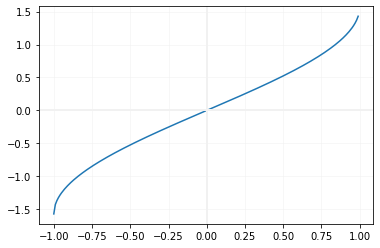# asin()

asin(x) returns arc sin of x. Input number is in radian
``````import math
print(math.asin(1))     # 1.5707963267948966
print(math.asin(0.56))  # 0.5943858000010622
print(math.asin(-0.56)) # -0.5943858000010622
print(math.asin(-1))    # -1.5707963267948966
print(math.asin(0))     # 0.0 ``````
Note that all the inputs are in radian.
For any value more than 1 or less than -1 , we will get ValueError
``````print(math.asin(1.01))
print(math.asin(-1.01))``````
Above code will generate error.

## Inputs in degree

We can convert radian value to degree and use the same
``````import math
in_degree = 57
print(math.asin(in_redian))``````
Output
``1.4691422654884898``

## Drawing graph of asin()

In our Trigonometric language asin is also know as arcsin. Using this we will use Matplotlib to generate graph of asin``````import matplotlib.pyplot as plt
x=[]
y=[]
i=-1
while (i<=1):
x.append(i)
y.append(math.asin(i))
i=i+0.01
plt.plot(x,y)
plt.show()``````

Subscribe to our YouTube Channel here

## Subscribe

* indicates required
Subscribe to plus2netplus2net.com